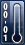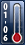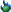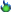1. ##Marble formula

I can't find the formula for how many 1 inch marbles there are in a 2 and 3 inch marble, but it is one of those fascinating things we should all know. Anyone know what it was?Reply With Quote

2.Glass-a-holicJoin Date
Aug 2014
Posts
1,919
Rep Power
27

##Re: Marble formula

1 inch is 0.524 CI
2 inch is 4.19 ci
3 inch is 14.14 ci

So a 3 inch marble has 26.98473 1 inch marbles in it.

Seems crazy don't it ? but that is what my math works out to be..

I just calculate the volume of a sphere then divide one to the other.Reply With Quote

3. ##Re: Marble formula

can you just use a scale?

initially i did agree that the numbers are somewhat mind boggling. After thinking for a second, i think it is more the shape that is boggling the brain. As a sphere it tricks the mind.

If you use the idea with a cube for instance it becomes clear very fast. A 1" cube could fit into a 3" cube 27 times. and that seems to come into focus rather easy vs a sphere...
Last edited by Mute; 04-29-2019 at 04:28 AM.Reply With Quote

4. ##Re: Marble formulaReply With Quote

5.Glass-a-holicJoin Date
Jul 2015
Location
Indiana
Posts
7
Rep Power
0

##Re: Marble formula

Hi Misha, if you are asking about the volume of a marble the answer is relatively simple if my algebra is correct. The formula for the volume of a sphere is 4/3*pi*r^3 or 1/6*pi*d^3 where r is the radius and d is the diameter. The ratio of a marble's volume to that of a 1" marble is then simply d^3 or d*d*d. So the volume of a 2" marble is 8 times that of a 1" marble and a 3" marble is 27 times that of a 1" marble. OF course the formula works for fractional diameters also. a 0.5" marble would have 1/8 the volume of a 1" marble. You can verify these number by measuring the volume water displaced by a 1" marble compared to the amount displaced be a larger or smaller marble. Instead of measuring the volume of water you could measure its weight. We all have gram scales don't we?Reply With Quote

6. ##Re: Marble formula

Grdunc is almost right, although they forgot to add the 1.3333 (4/3 in decimals) and the value for Pi into the final equation - normally 3.14 for quick, on-the-fly calculations.

So, a marble with a diameter of 1" = (1.33)*(3.14)*[(1)*(1)*(1)]= 4.19 cubic inches.
And a marble with a diameter of 2"= (1.33)*(3.14)*[(2)*(2)*(2)]= 33.51 cubic inches.

If you fancy messing about with the formula and putting in some different marble diameters, here's a handy formula page. https://www.calculatorsoup.com/calcu...ids/sphere.php

Hope this helps! I thought basic numeracy and statistics to adults for a decade, before moving on - so I'm glad to get to use those skills again :-)Reply With Quote

7.Glass-a-holicJoin Date
Aug 2014
Posts
1,919
Rep Power
27

##Re: Marble formulaOriginally Posted by SpeedingpulletGrdunc is almost right, although they forgot to add the 1.3333 (4/3 in decimals) and the value for Pi into the final equation - normally 3.14 for quick, on-the-fly calculations.

So, a marble with a diameter of 1" = (1.33)*(3.14)*[(1)*(1)*(1)]= 4.19 cubic inches.
And a marble with a diameter of 2"= (1.33)*(3.14)*[(2)*(2)*(2)]= 33.51 cubic inches.

If you fancy messing about with the formula and putting in some different marble diameters, here's a handy formula page. https://www.calculatorsoup.com/calcu...ids/sphere.php

Hope this helps! I thought basic numeracy and statistics to adults for a decade, before moving on - so I'm glad to get to use those skills again :-)
You might want to run the numbers again. Those look way off.Reply With Quote

8. ##Re: Marble formulaReply With Quote

9.Glass-a-holicJoin Date
Oct 2017
Posts
5
Rep Power
0

##Re: Marble formula

Its funny how t adds upReply With Quote

10. ##Re: Marble formula

So basically there are about 27 x 1" marbles in a 3" marble. Sounds about right to me when I hold one or the other in my hand. It's still mind boggling though haha. Thanks so much you guys! And sorry it took me a bit to reply. I had a huge cardiac exam, which believe it or not, uses a ton of math. Which I suck at. I still got a 94.69. woot.Reply With Quote

11.Glass-a-holicJoin Date
Aug 2017
Posts
9
Rep Power
0

##Re: Marble formula

Download the marble calculator app in the google play store. You can choose the mm rod you want to use and it tells you the length you need to make itReply With Quote

####Posting Permissions

• You may not post new threads
• You may not post replies
• You may not post attachments
• You may not edit your posts
•

Featured Member's Spotlight!Donate to our forum!